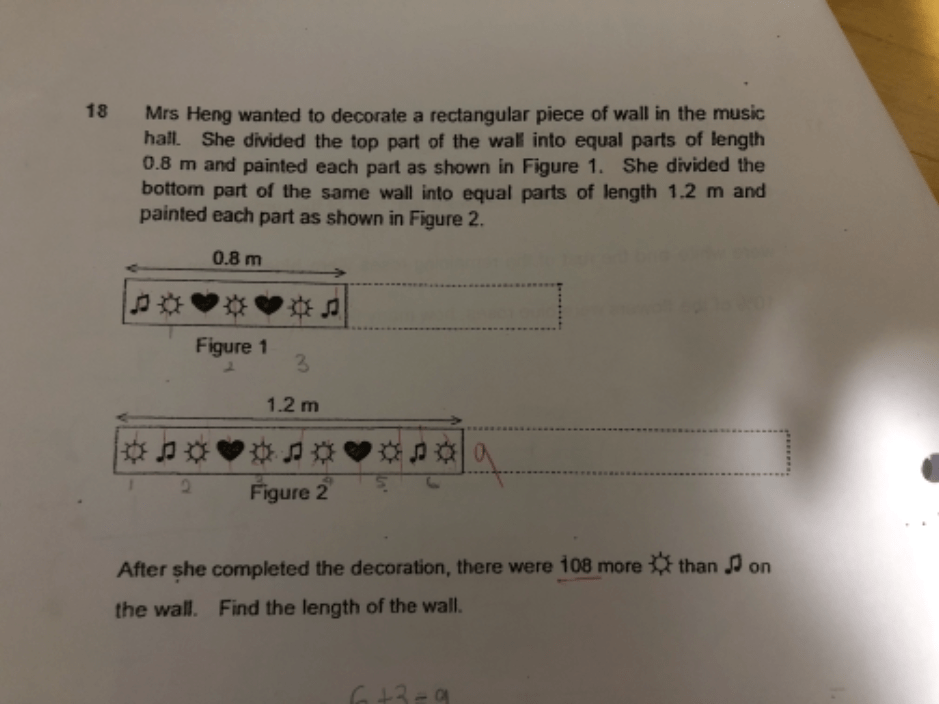# QuestionAppreciate any help on this length question.

thanks

Source: Nanyang Primary

LCM of 8 and 12 = 24
In every 2.4 m —
top part of wall had: 3 x 3 = 9 (sun signs) and 2 x 3 = 6 (music signs)
bottom part of wall had : 6 x 2 = 12 (sun signs) and 3 x 2 = 6 (music signs)
9 + 12 – 6 – 6 = 9 (more sun signs in every 2.4 m)
108/9 = 12
12 x 2.4 = 28.8

Ans : 28.8 m.

2 Replies 1 Like ✔Accepted Answer

thanks appreciate the highlight of using LCM